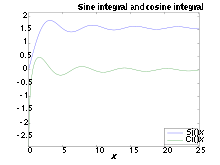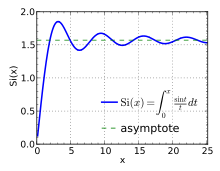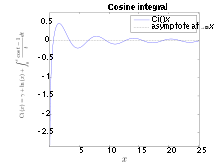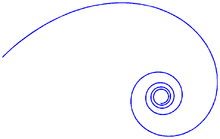# Trigonometric integral

﻿
Trigonometric integralSi(x) (blue) and Ci(x) (green) plotted on the same plot.

In mathematics, the trigonometric integrals are a family of integrals which involve trigonometric functions. A number of the basic trigonometric integrals are discussed at the list of integrals of trigonometric functions.

## Sine integralPlot of Si(x) for 0 ≤ x ≤ 8π.

The different sine integral definitions are:${\rm Si}(x) = \int_0^x\frac{\sin t}{t}\,dt$${\rm si}(x) = -\int_x^\infty\frac{\sin t}{t}\,dt$

Si(x) is the primitive of sinx / x which is zero for x = 0; si(x) is the primitive of sinx / x which is zero for$x=\infty$.

Note that$\frac{\sin t}{t}$ is the sinc function and also the zeroth spherical Bessel function.

When$x=\infty$, this is known as the Dirichlet integral.

In signal processing, the oscillations of the Sine integral cause overshoot and ringing artifacts when using the sinc filter, and frequency domain ringing if using a truncated sinc filter as a low-pass filter.

The Gibbs phenomenon is a related phenomenon: thinking of sinc as a low-pass filter, it corresponds to truncating the Fourier series, which causes the Gibbs phenomenon.

## Cosine integralPlot of Ci(x) for 0 < x ≤ 8π.

The different cosine integral definitions are:${\rm Ci}(x) = \gamma + \ln x + \int_0^x\frac{\cos t-1}{t}\,dt$${\rm ci}(x) = -\int_x^\infty\frac{\cos t}{t}\,dt$${\rm Cin}(x) = \int_0^x\frac{1-\cos t}{t}\,dt$

ci(x) is the primitive of cos x / x which is zero for$x=\infty$. We have:${\rm ci}(x)={\rm Ci}(x)\,$${\rm Cin}(x)=\gamma+\ln x-{\rm Ci}(x)\,$

## Hyperbolic sine integral

The hyperbolic sine integral:${\rm Shi}(x) = \int_0^x\frac{\sinh t}{t}\,dt = {\rm shi}(x).$

## Hyperbolic cosine integral

The hyperbolic cosine integral:${\rm Chi}(x) = \gamma+\ln x + \int_0^x\frac{\cosh t-1}{t}\,dt = {\rm chi}(x)$

where γ is the Euler–Mascheroni constant.

## Nielsen's spiralNielsen's spiral.

The spiral formed by parametric plot of si,ci is known as Nielsen's spiral. It is also referred to as the Euler spiral, the Cornu spiral, a clothoid, or as a linear-curvature polynomial spiral. The spiral is also closely related to the Fresnel integrals. This spiral has applications in vision processing, road and track construction and other areas.

## Expansion

Various expansions can be used for evaluation of Trigonometric integrals, depending on the range of the argument.

### Asymptotic series (for large argument)${\rm Si}(x)=\frac{\pi}{2} - \frac{\cos x}{x}\left(1-\frac{2!}{x^2}+\cdots\right) - \frac{\sin x}{x}\left(\frac{1}{x}-\frac{3!}{x^3}+\cdots\right)$${\rm Ci}(x)= \frac{\sin x}{x}\left(1-\frac{2!}{x^2}+\cdots\right) -\frac{\cos x}{x}\left(\frac{1}{x}-\frac{3!}{x^{3}}+\cdots\right)$

These series are divergent[clarification needed], although can be used for estimates and even precise evaluation at$~{\rm Re} (x) \gg 1~$.

### Convergent series${\rm Si}(x)= \sum_{n=0}^\infty \frac{(-1)^{n}x^{2n+1}}{(2n+1)(2n+1)!}=x-\frac{x^3}{3!\cdot3}+\frac{x^5}{5!\cdot5}-\frac{x^7}{7! \cdot7}\pm\cdots$${\rm Ci}(x)= \gamma+\ln x+\sum_{n=1}^{\infty}\frac{(-1)^{n}x^{2n}}{2n(2n)!}=\gamma+\ln x-\frac{x^2}{2!\cdot2}+\frac{x^4}{4! \cdot4}\mp\cdots$

These series are convergent at any complex$~x~$, although for$|x|\gg 1$ the series will converge slowly initially, requiring many terms for high precision.

## Relation with the exponential integral of imaginary argument

Function${\rm E}_1(z) = \int_1^\infty \frac {\exp(-zt)} {t} {\rm d} t \qquad({\rm Re}(z) \ge 0)$ is called exponential integral. It is closely related with Si and Ci:${\rm E}_1( {\rm i}\!~ x)= i\left(-\frac{\pi}{2} +{\rm Si}(x)\right)-{\rm Ci}(x) = i~{\rm si}(x) - {\rm ci}(x) \qquad(x>0)$

As each involved function is analytic except the cut at negative values of the argument, the area of validity of the relation should be extended to Re(x) > 0. (Out of this range, additional terms which are integer factors of π appear in the expression).

Cases of imaginary argument of the generalized integro-exponential function are$\int_1^\infty \cos(ax)\frac{\ln x}{x}dx = -\frac{\pi^2}{24}+\gamma(\frac{\gamma}{2}+\ln a)+\frac{\ln^2a}{2} +\sum_{n\ge 1}\frac{(-a^2)^n}{(2n)!(2n)^2},$

which is the real part of$\int_1^\infty e^{iax}\frac{\ln x}{x}dx = -\frac{\pi^2}{24} +\gamma(\frac{\gamma}{2}+\ln a)+\frac{\ln^2 a}{2}-\frac{\pi}{2}i(\gamma+\ln a) +\sum_{n\ge 1}\frac{(ia)^n}{n!n^2}.$

Similarly$\int_1^{\infty} e^{iax}\frac{\ln x}{x^2}dx =1+ia[-\frac{\pi^2}{24}+\gamma(\frac{\gamma}{2}+\ln a-1)+\frac{\ln^2 a}{2}-\ln a+1 -\frac{i\pi}{2}(\gamma+\ln a-1)]+\sum_{n\ge 1}\frac{(ia)^{n+1}}{(n+1)!n^2}.$

### Signal processing

Wikimedia Foundation. 2010.

### Look at other dictionaries:

• Integral — This article is about the concept of integrals in calculus. For the set of numbers, see integer. For other uses, see Integral (disambiguation). A definite integral of a function can be represented as the signed area of the region bounded by its… …   Wikipedia

• Trigonometric substitution — In mathematics, trigonometric substitution is the substitution of trigonometric functions for other expressions. One may use the trigonometric identities to simplify certain integrals containing radical expressions:: ext{For } sqrt{a^2 x^2} ext{… …   Wikipedia

• Trigonometric functions — Cosine redirects here. For the similarity measure, see Cosine similarity. Trigonometry History Usage Functions Generalized Inverse functions …   Wikipedia

• trigonometric series — noun : a mathematical series whose terms proceed by sines and cosines of integral multiples of a variable angle * * * Math. an infinite series involving sines and cosines of increasing integral multiples of a variable. * * * trigonometric series …   Useful english dictionary

• trigonometric series — Math. an infinite series involving sines and cosines of increasing integral multiples of a variable. * * * …   Universalium

• List of integrals of trigonometric functions — The following is a list of integrals (antiderivative functions) of trigonometric functions. For integrals involving both exponential and trigonometric functions, see List of integrals of exponential functions. For a complete list of Integral… …   Wikipedia

• List of trigonometric identities — Cosines and sines around the unit circle …   Wikipedia

• Multiple integral — Topics in Calculus Fundamental theorem Limits of functions Continuity Mean value theorem Differential calculus  Derivative Change of variables Implicit differentiation Taylor s theorem Related rates …   Wikipedia

• Elliptic integral — In integral calculus, elliptic integrals originally arose in connection with the problem of giving the arc length of an ellipse. They were first studied by Giulio Fagnano and Leonhard Euler. Modern mathematics defines an elliptic integral as any… …   Wikipedia

• Inverse trigonometric functions — Trigonometry History Usage Functions Generalized Inverse functions Further reading …   Wikipedia